Remainder Theoram

Chapter 2 Class 9 Polynomials
Concept wise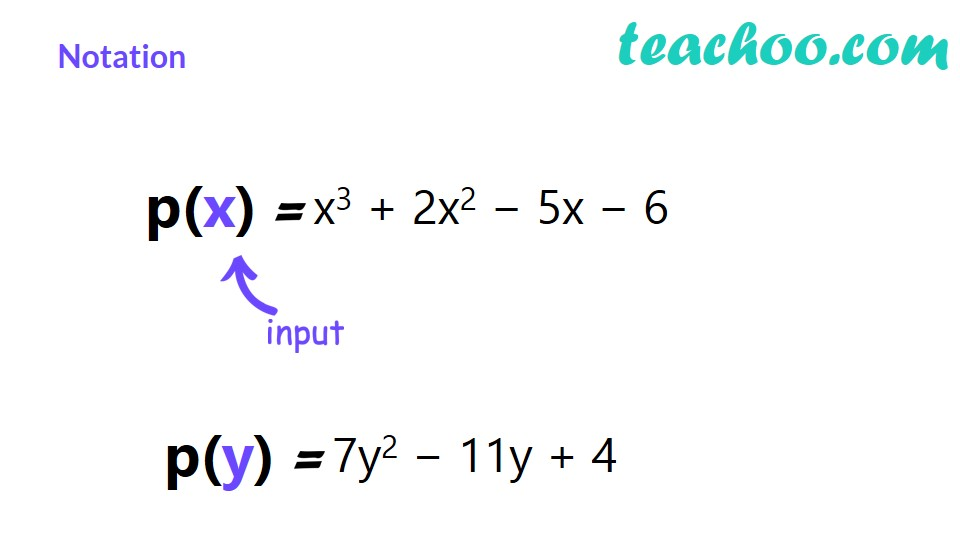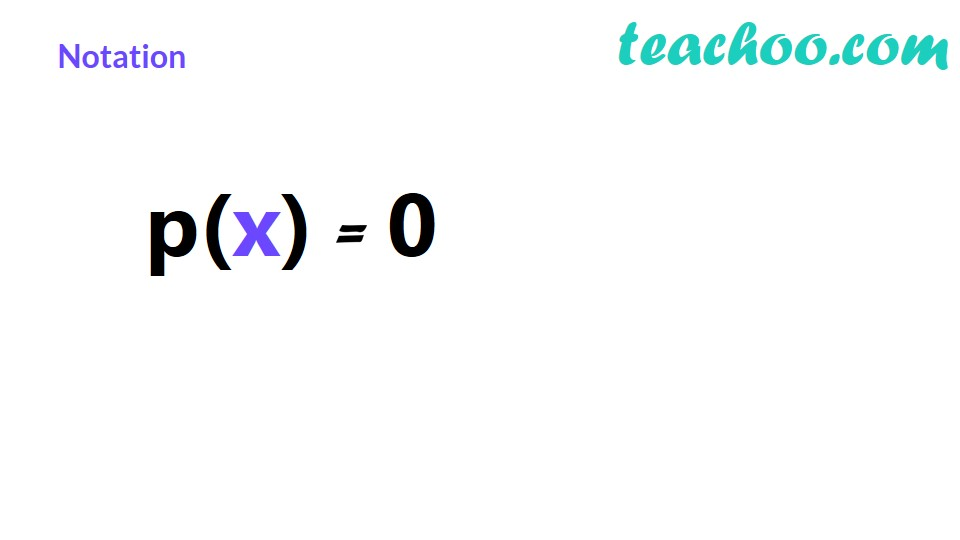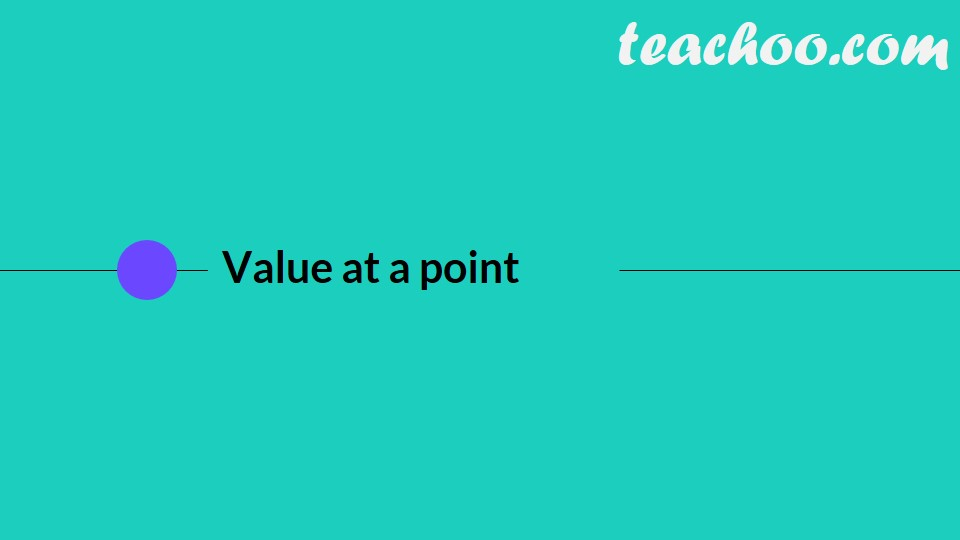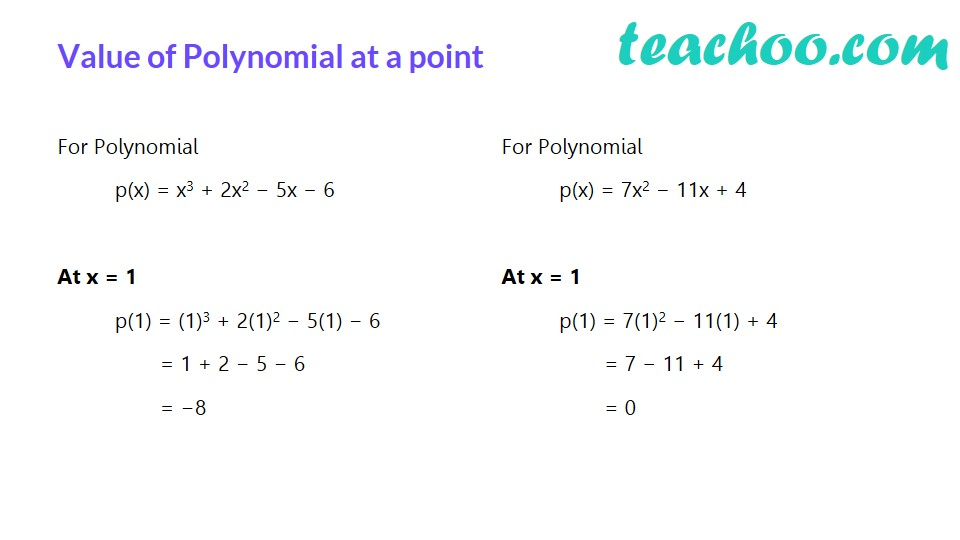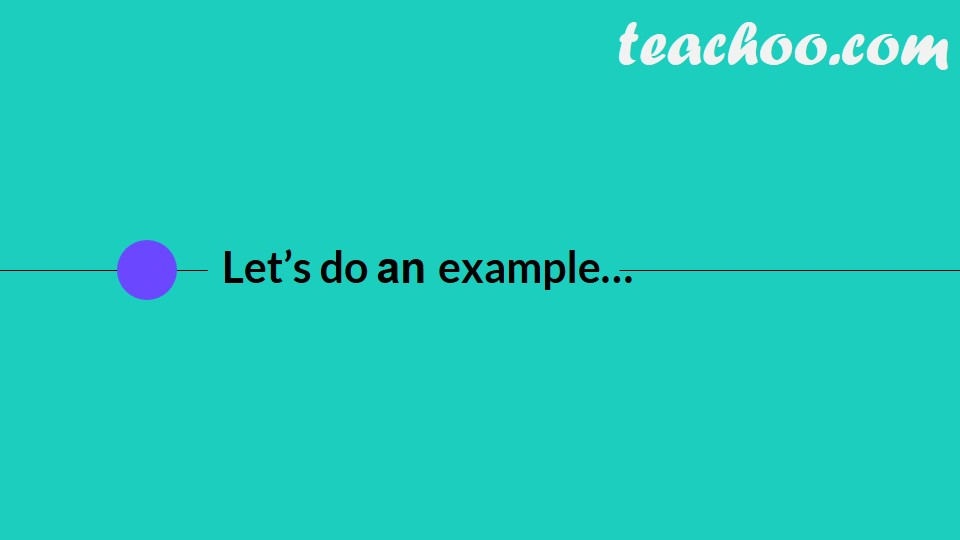Let us first divide 7 by 3,

7/3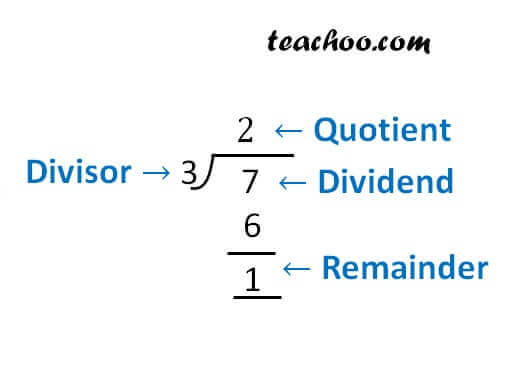Here

7 = 3 × 2 + 1

i.e.  Dividend = Divisor × Quotient + Remainder

We can do the same when dividing two polynomials

In Example 6 ,

We divided  3x 2 + x − 1 by 1 + x

Our  division was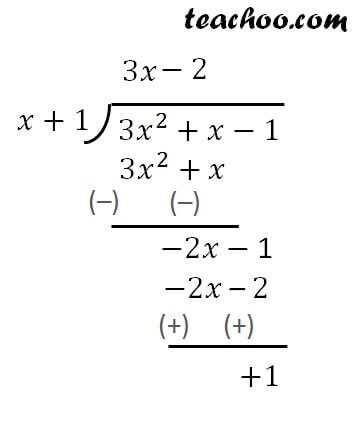Here

Dividend = 3x 2 + x – 1

Divisor = x + 1

Quotient = 3x – 2

And Remainder = 1

Dividend = Divisor × Quotient + Remainder

3x 2 + x – 1 = (x + 1) (3x – 2) + 1

3x 2 + x – 1 = x (3x – 2) + 1 (3x – 2) + 1

3x 2 + x – 1 = 3x 2 – 2x + 3x – 2 + 1

3x 2 + x – 1 = 3x 2 + x – 1

Get live Maths 1-on-1 Classs - Class 6 to 12

### Transcript

Dividing two numbersQuotient Divisor Dividend Remainder Which can be rewritten as a sum like this: Division Algorithm is Dividend = Divisor × Quotient + Remainder Quotient Divisor Dividend Remainder Dividing two Polynomials Let’s divide 3x2 + x − 1 by 1 + x We can write Dividend = Divisor × Quotient + Remainder 3x2 + x – 1 = (x + 1) (3x – 2) + 1 What if…We don’t divide? We divide 3x2 + x − 1 by x + 1 So, Dividend = Divisor × Quotient + Remainder 3x2 + x – 1 = (x + 1) × Quotient + Remainder Putting x = −1 3(−1)2 + (−1) – 1 = (−1 + 1) × Quotient + Remainder 1 = Remainder Remainder = 1